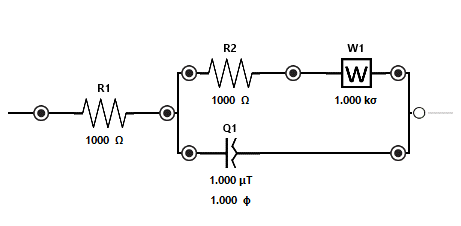# Electrochemistry and Aptamers IV

Electrical impedance spectroscopy is an approach to measuring the modulation of the surface of an electrode as a result of the binding of a target molecule with an aptamer without labeling the aptamer with a redox moiety such as methylene blue. In this approach we measure impedance as a function of current through an electrical system. Impedance is however a complex number, that is it has both an imaginary and a real component.

We can think of the electrode in buffer system as an electrical cell. This takes us to the image we used at the start of this blog. This is a schematic diagram of an applicable electrical system. We have two resistors (R1 and R2), a Warburg element (W1) and a constant phase element (Q1). Don’t worry, this will start to make sense now very soon.

R1 =  the resistance of the electrode.

R2 = the resistance on the interface of the electrode and the buffer.

W1 = is a measurement of diffusion through the buffer

Q1 = is a constant phase element (this is a measure of system capacitance).

We find that using a model with Q1 rather than a straight capacitor results in better fits to our system. In model fitting, Q1 is composed of two variables, Q1, the capacitance activity, and n1 which is an indication of how much the system is acting as a capacitor versus a resistor.

R2 is the element that we are primarily interested in, although Q1 is also of interest. Software exists to map the response over a range of frequencies to estimate the relative values for each of these components to obtain a best fit to the observed data. From this we get a direct estimate of the resistance (R2) on the surface of the electrode.

DNA itself is negatively charged and this increases the resistance of the system. Measurements with EIS are performed with the following steps. First, we measure the baseline for the electrode in redox buffer. Then we add the appropriate target sample in binding buffer to the electrode and let that incubate for a few minutes. Then this is removed and replaced with redox buffer and a “with target” measurement is performed. There is a lot of chip to chip variability with commercially available chips so we rely on the measurement with target divided by the baseline measurement as our key statistic. I like to subtract one from this division to give us a clear idea of the direction of response. The binding of a target molecule increases resistance.

The primary advantages of this approach are the high level of sensitivity possible. The key disadvantages are variability in chip to chip measurements and the time required per measurement. We are actively working with this approach, and we are continuing to gain insights into how best to improve performance. Sometimes this work does feel like going up the stairs against the flow. We welcome you to share your insights with this approach. Those of us going against the flow, need to work together.

neoaptamers